• 其中是协方差矩阵协方差矩阵的逆可以记作，也叫信息矩阵。当变量xx是三维变量时，协方差矩阵为： 其中 其实在应用中，往往我们直接操作的是信息矩阵，而不是协方差矩阵。下面从一个例子来体会一下协方差矩阵...
零均值的多元高斯分布有如下概率形式：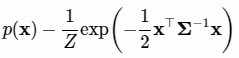其中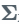是协方差矩阵，协方差矩阵的逆可以记作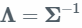，也叫信息矩阵。当变量xx是三维变量时，协方差矩阵为：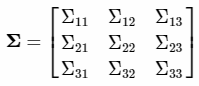其中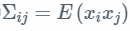其实在应用中，往往我们直接操作的是信息矩阵，而不是协方差矩阵。下面从一个例子来体会一下协方差矩阵与信息矩阵。

example

假设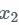为室外的温度，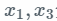分别是房间1与房间3的室内温度：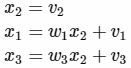其中，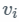为相互独立，且各自服从协方差为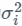的高斯分布。根据上面它们之间的联系，我们可以求出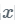的协方差矩阵，首先：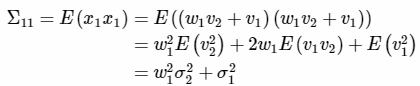然后同理，可以求出另外两个对角元素为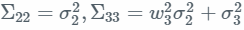。而对于协方差矩阵的非对角元素有：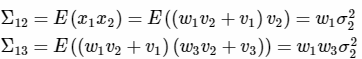依次类似，可以得到完整的协方差矩阵为：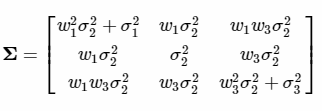信息矩阵是协方差矩阵的逆矩阵，此处我们可以通过计算联合高斯分布来得到协方差矩阵的逆：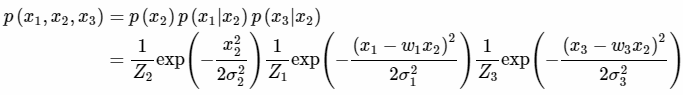利用指数性质，可以计算出联合概率分布如下：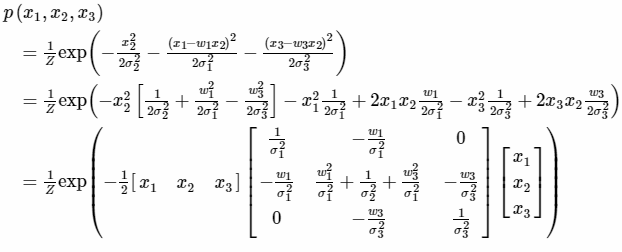所以，这里上面矩阵就是协方差矩阵的逆，也就是信息矩阵：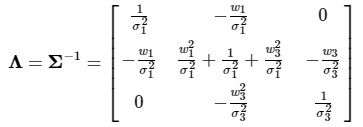由上，可以看到当在协方差矩阵中，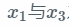之间是相关的，而在信息矩阵中，它们是相互独立的（相关系数为0）,这是因为，我们在推导信息矩阵时是使用了联合分布的链式法则，信息矩阵中的相关性在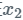确定之后计算的，此时它们是相互独立的。

上述例子中去掉x3

协方差矩阵直接只计算前两个相关的协方差矩阵即可，也就是去掉划线的部分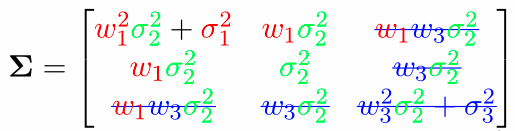变为：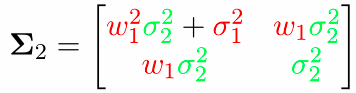至于信息矩阵，只需要把信息矩阵公式中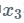相关的部分（蓝色）去掉：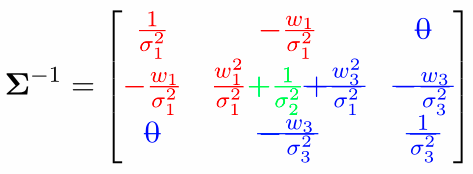得到：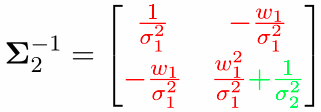之所以要移除一个变量，然后再算它的信息矩阵，是因为在实际应用中经常会用到这样的操作，上面只讲了原理，下面会抽时间讲讲如何快速实现，会需要舒尔补(Schur’s complement)与边缘化(marginalization)。

补充

此处需要注意，协方差矩阵与信息矩阵都可以用来表示多元变量之间的相关性。但是，协方差矩阵是衡量的变量之间边界概率关系，通常是直接相关性，信息矩阵会有间接相关性，衡量的是变量之间的条件概率关系。因此，在计算中我们会发现有些协方差矩阵中相关的两个量在信息矩阵中不相关，有些协方差矩阵中不相关的两个量在信息矩阵中相关。

比如在下面这个例子中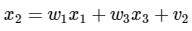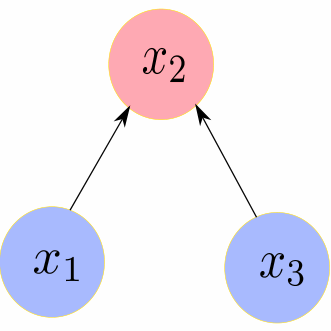协方差矩阵为：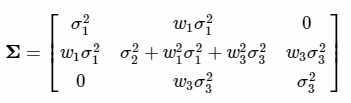信息矩阵为：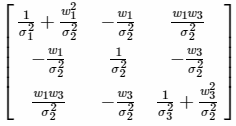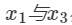在协方差矩阵中不相关，在信息矩阵中相关。这是因为协方差矩阵中是直接相关性，信息矩阵可以使用链式法则推导，其中当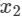固定后，这两个变量就会变成相关的了。


展开全文• 协方差矩阵 对于一维随机变量直接用方差即可衡量随机变量x与其期望E(x)的偏离程度，对于多维随机变量X，需要用一个矩阵来表示偏离程度，矩阵的对角线是每个维度自己的方差，对角线以外表示不同的维度之间的协方差，...
协方差矩阵

对于一维随机变量直接用方差即可衡量随机变量x与其期望E(x)的偏离程度，对于多维随机变量X，需要用一个矩阵来表示偏离程度，矩阵的对角线是每个维度自己的方差，对角线以外表示不同的维度之间的协方差，所以协方差矩阵是实对称矩阵。

协方差矩阵的计算公式

所以有如下性质：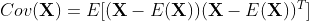如果随机向量Y=PX，其中X，Y为随机向量，P为矩阵（方阵）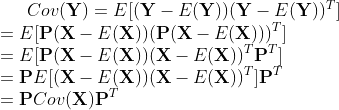也就是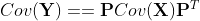多元高斯分布

一元高斯分布概率密度函数如下：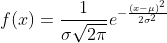多元高斯分布为：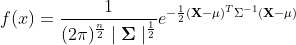具体推导过程可以参考：https://zhuanlan.zhihu.com/p/36522776

https://zhuanlan.zhihu.com/p/58987388

主要的更改是把方差换成了协方差矩阵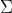，2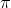的次方换成n次，n为X向量的维数。

如果协方差矩阵是对角阵，则X向量的每一维彼此独立，互相概率不影响，高维的不好想象可以想象二维的，此时X随机变量的概率密度函数是一个立体的高斯，其平行于坐标平面的截面是一个圆。

如果协方差矩阵除对角线外其他位置为非零，则X向量存在彼此不独立的维，拿二维举例，此时X随机变量的概率密度函数是一个立体的高斯，其平行于坐标平面的截面是一个椭圆，且椭圆的长轴不与坐标轴平行，即椭圆是倾斜的。

根据线性代数的知识可知，由于协方差矩阵为实对称矩阵，所以必定可以用单位正交矩阵相似对角化，根据Y=PX，则，可知，只需要求出协方差矩阵的特征值，并进一步求出单位正交矩阵P，就可以将X向量转换为Y向量，此时Y向量的协方差矩阵Cov(Y)就是经过Cov(X)相似对角化所得，所以是对角阵，所以Y向量的每个维度相互独立，也就是X向量可以经过线性变换由每个维度相互不独立转换成相互独立，对于二维来说，就是概率密度函数的截面由倾斜的椭圆转换成不倾斜的椭圆。

另外协方差矩阵为非负定矩阵，也就是半正定阵（正定的定义见https://blog.csdn.net/xiaoyink/article/details/103059397），证明如下：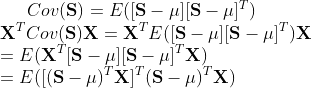第二行左边乘以一个向量的转置，右边乘以一个向量，是参考正定的定义来的，只要证明其结果大于等于0，即为半正定阵，

最后的结果中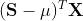是一个数，转置之后还是一个数，所以相当于求一个数平方的期望，肯定是大于等于0的，所以为半正定阵。

展开全文• 协方差矩阵的几何解释和多重高斯分布 这篇文章，总结之前阅读的这篇文章学到的知识点，即协方差矩阵的几何意义，同时利用几何解释，介绍多重高斯分布如何从标准单变量的高斯分布推导出来。主要涉及如下内容： ...
协方差矩阵的几何解释和多重高斯分布

这篇文章，总结之前阅读的这篇文章学到的知识点，即协方差矩阵的几何意义，同时利用几何解释，介绍多重高斯分布如何从标准单变量的高斯分布推导出来。主要涉及如下内容：

协方差矩阵的定义
协方差矩阵所体现的数据分布的几何意义
多重高斯分布的推导

协方差矩阵的定义
协方差矩阵一般出现在多重高斯分布的表达式中：
$p(x;\mu,\Sigma)=\frac{1}{(2\pi)^{\frac{n}{2}}|\Sigma|^{\frac{1}{2}}}exp(-\frac{1}{2}(x-\mu)^T\Sigma^{-1}(x-\mu)) \tag{1}$
其中$\mu$是列向量$x$的平均值构成的向量，而$\Sigma$即协方差矩阵。对于任意列向量来$Z$来说，其协方差矩阵的定义为：
$Cov(Z)=E[(Z-E(Z))(Z-E(Z)^T] \\ =E[ZZ^T]-(E[Z])(E[Z])^T \tag{2}$
列向量减去自身的均值向量，而后和自己的转置相乘，将会得到一个矩阵，参考两个变量之间的协方差的定义：
$Cov(x,y)=E[(x-E(x))(y-E(y))] \tag{3}$
可以知道，得到的矩阵的第$c_{ij}$个变量就是第$i$维的变量和第$j$维的变量的协方差，而且$c_{ij}=c_{ji}$，所以这个矩阵是对称的，而且由这个矩阵的变换功能本身来看，也必定至少是半正定的(positive semi-definite)。
对等式2来说，之所以等于$E(ZZ^T)-(E[Z])(E[Z)])^T$是基于矩阵的第$ij$个元素有：
$\sigma(z_i,z_j)=E[(z_i-E(z_i))(z_j-E(z_j))]\\ =E[z_iz_j-E(z_i)z_j-E(z_j)z_i+E(z_i)E(z_j)] \\ =E[z_iz_j]-\mu_iE(z_j)-\mu_jE(z_i)+\mu_i\mu_j\\ =E[z_iz_j]-\mu_i\mu_j \tag{4}$
所以可得到等式2
期望和方差也有值得留意的两个性质，比如，如果随机变量$X$和$Y$相互独立，那么：
$E(XY)=\Sigma_i\Sigma_jx_iy_jp(x_i,y_j)\\ =\Sigma_ix_ip(x_i)\Sigma_jy_jp(y_j)\\ =E(X)E(Y) \tag{5}$
对方差来说：
$Var(X)=E[(x-\mu)^2]=\Sigma_x(x-\mu)^2p(x)\\ =\Sigma_x(x^2-2x\mu+\mu^2)p(x)\\ =\Sigma_xx^2p(x)-\Sigma_x2\mu xp(x)+\mu^2\\ =\Sigma_xx^2p(x)-\mu^2\\ =E(x^2)-E(x)^2 \tag{6}$
上边的等式5和6这里列出来只是复习一下，让我们看到方差和期望的一些性质
其实单从协方差矩阵，我们没有办法有什么过于深入的认识，对于一维的高斯分布来说，比如：我们可以看到，均值就是高斯分布中心的位置，而方差则描述了数据分布离均值的情况，方差越大，表面数据相对于中心点越是分散，越小，表明离中心点越是密集，所以方差用来描述数据离开中心均值的离散程度
如果随机变量服从高斯分布，而且均值已知，那么方差的估计值为：
$\sigma=\frac{1}{N}\Sigma(x-\mu)^2 \tag{7}$
如果均值$\mu$和方差均为估计值，那么此时：
$\mu=\frac{\Sigma x}{N} \tag{8}$
而方差的无偏估计则是：
$\sigma=\frac{1}{N-1}\Sigma(x-\mu)^2 \tag{9}$
之所以方差在两种情况下有差异，一个是除以$N$，一个是除以$N-1$，这个和在假定变量为高斯分布的时候，均值是否可知有关，详情可以参考这篇文章
那多重高斯分布所对应的协方差矩阵又有什么意义呢？
协方差矩阵所体现的数据分布的几何意义
通过协方差的定义和协方差的组织形式我们可以知道，协方差描述了变量数据在各个方向上的分布，比如如下所示就是二维的数据点的一个分布：$x$和$y$方向的数据成正相关分布，因为$x$增大，$y$也跟着具有这种趋势，这个数据的分布情况，可以用协方差矩阵表示：
$\Sigma = \begin{bmatrix} \\ \sigma(x,x) & \sigma(x,y) \\ \sigma(y,x) & \sigma(y,y) \\ \end{bmatrix} \tag{10}$
对于不同的二维协方差矩阵，数据分布：矩阵的特征值分解
通过看这些图的数据分布情况，隐约感觉到了一种趋势，实际上对于矩阵来说，特别是对称的方阵，通常我们可以进行特征值分解，而特征向量的方向，往往就是矩阵变换作用的方向。一个矩阵乘以一个向量，其实就是把向量投影到自身的列空间中，对于方阵而言，乘以一个向量，可以把向量投影到各个特征向量方向分别计算然后组合形成最终的结果，所以特征向量描述了矩阵变换的特性。
对于标准单位向量$v$而言，最大化$v^T\Sigma v$的值的问题，可以被转化为求解瑞利商(Rayleigh Quotient)，其最大的方向$v$就是矩阵$\Sigma$的具有最大特征值的特征向量方向。
由于一个变换矩阵，可以由其特征向量和特征值完整描述，应用到协方差矩阵来说，这意味着:
$\Sigma v=\lambda v \tag{11}$
其中$v$为特征向量，$\lambda$为特征值
如果协方差矩阵为对角矩阵，那么这个矩阵的主要功能就是把各个坐标分量进行拉伸，比如：但是如果协方差矩阵不是对角矩阵，那么数据分布则会类似于下图这样：对比上下两个图，虽然不是对角矩阵的情况更为复杂，但是从趋势来说，下图只是上图旋转后的结果
如果我们把变换矩阵$T$定义为：
$T=RS \tag{12}$
其中$R$为旋转矩阵，比如：
$R = \begin{bmatrix}\\ cos(\theta) & -sin(\theta) \\ sin(\theta) & cos(\theta) \\ \end{bmatrix} \tag{13}$
$S$为拉伸矩阵，比如：
$S = \begin{bmatrix}\\ s_1 & 0\\ 0 & s_2\\ \end{bmatrix} \tag{14}$
且$s_1$和$s_2$分别为两个方向上的拉伸因子
那么协方差矩阵其实可以被这样拆解成两个变换成分，一个是拉伸变换成分，一个是旋转变换成分，我们可以通过依次应用这两个成分得到任意协方差矩阵
比如，如下所示是高斯白噪声对应的二维数据分布：其对应的协方差矩阵是：
$\Sigma = \begin{bmatrix}\\ 1 & 0\\ 0 & 1\\ \end{bmatrix}$
这个图像，很好的体现了高斯白噪声的数据分布，此时方差为1，均值为0，这个时候，如果我们让各个数据点乘以拉伸矩阵呢？此时：
$x^{'}=Sx$
如果
$S = \begin{bmatrix}\\ 5 & 0\\ 0 & 1\\ \end{bmatrix}$
那么原先白噪声高斯分布的图像就会变成这样：可以看到，数据点在$x$方向上拉伸了，这样可以知道，拉伸矩阵的作用就是让各个数据点在各个放下上更加分散，这样方差也就对应的大了
此时再应用旋转矩阵，可以得到
$y = Rx^{'}$
那么最终的图像就会变成数据分布一般的情况：所以
$y = RSx$
计算变换后的协方差矩阵的时候，由于均值为0，其实就是：
$Cov(Y)=E[(RSx)(RSx)^T]=E[RSxx^TS^TR^T]\\ =RSE[xx^T]S^TR^T=RSISR^T\\ =RS^2R^T\tag{15}$
上边由于$xx^T$是一个数，所以可以提取出来，而且$R$是标准正交的矩阵，$S$是对角矩阵
可以看到，对原始白噪声数据变换后的结果，求取协方差矩阵只会包含旋转变换矩阵和拉伸矩阵
多重高斯分布的推导
其实上边这种先从标准数据，然后应用变换的思路，可以应用于多重高斯分布的表达式的推导
对于一维的标准正态分布来说，其表达式等于：
$p(x;0,1)=\frac{1}{(2\pi)^{\frac{1}{2}}}exp(-\frac{x^2}{2}) \tag{16}$
对于多重变量的标准正态分布，由于各个变量之间相互独立，所以联合概率密度等于：
$p(x_1,x_2,...,x_n;0,1)=p(x_1;0,1)p(x_2;0,1)...p(x_n;0,1)\\ =\frac{1}{(2\pi)^{\frac{n}{2}}}exp(-\frac{\Sigma_ix_i^2}{2})\\ =\frac{1}{(2\pi)^{\frac{n}{2}}}exp(-\frac{x^Tx}{2}) \tag{17}$
可以看到，由于均值为0，且各个方向上的方差均为1，还是比较均匀的，这个时候，如果想要生成通常情况下的高斯分布，那么我们可以像上一小节那样，把标准的$x$向量进行旋转拉伸变换，然后加上平移向量进行平移变换，这个时候有
$y=RSx+\mu \tag{18}$
所以，我们可以得到
$x=(RS)^{-1}(y-\mu) \tag{19}$
结合概率密度的积分函数，我们知道：
$1=\int...\int p(x_1,...,x_n)dx\\ =\int...\int \frac{1}{(2\pi)^{\frac{n}{2}}}exp(-\frac{x^Tx}{2}) dx \tag{20}$
对于多重变量微积分来说，由于有等式18的关系，所以微元有：
$dy=|RS|dx \tag{21}$
这里边的微元是对于向量的微元，可以认为$dx=dx_1dx_2...dx_n$，而$|RS|$则是求解行列式，行列式的几何意义就是方阵的行/列向量所构成的超平行多面体的有向体积，具有线性关系的向量之间的变换的微元的体积之间的比例因子，就是这个变换方阵的行列式，这一点涉及多变量微积分，这里我也不算是很清楚，不过有了等式21和19后，带入20即可得到：
$1=\int...\int p(x_1,...,x_n)dx\\ =\int...\int \frac{1}{(2\pi)^{\frac{n}{2}}}exp(-\frac{x^Tx}{2})dx \\ =\int...\int \frac{1}{(2\pi)^{\frac{n}{2}}|RS|}exp(-\frac{((RS)^{-1}(y - \mu))^T((RS)^{-1}(y - \mu))})dy\\ = \int...\int \frac{1}{(2\pi)^{\frac{n}{2}}|RS|}exp(\frac{(y-\mu)^{T}(S^{-1}R^{-1})^T(S^{-1}R^{-1})(y - \mu)}{2})dy\\ = \int...\int \frac{1}{(2\pi)^{\frac{n}{2}}|RS|}exp(\frac{(y-\mu)^{T}RS^{-1}S^{-1}R^T(y - \mu)}{2})dy \tag{22}$
这里边利用了$S$为对角矩阵，所以$S^T=S$，其逆矩阵也是如此，而$R$为旋转标准正交矩阵，所以$R^{-1}=R^T$，这个时候，如果$\Sigma=RSSR^T$，那么
$\Sigma^{-1}=RS^{-1}S^{-1}R^T$
$|RS|=|\Sigma|^{\frac{1}{2}}$
所以带入等式22就可以得到等式1中的多重高斯分布了，此时多重高斯分布之所以是这个形式的原因就非常明显了！
参考资料
1. A geometric interpretation of the covariance matrix
@fsfzp888
2019 年 04月


展开全文机器学习 线性代数 参数估计 数理统计
• 协方差矩阵是一个对称矩阵，决定了二维高斯分布的形状。 1.协方差矩阵的对角线元素为x和y轴的方差  2.反斜对角线上的两个值为协方差，表明x和y的线性相关程度（正值时：x增大，y也随之增大；负值时：x增大，y随之...
多维高斯分布：

协方差矩阵是一个对称矩阵，决定了二维高斯分布的形状。

1.协方差矩阵的对角线元素为x和y轴的方差
2.反斜对角线上的两个值为协方差，表明x和y的线性相关程度（正值时：x增大，y也随之增大；负值时：x增大，y随之减小）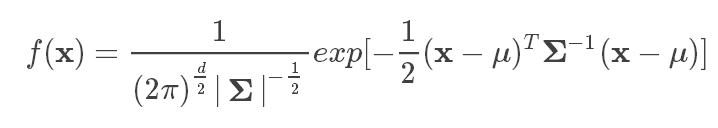展开全文• % Plot the first dataset (case #1) m=[0 0]'; S=[1 0;0 1]; N=500; X = mvnrnd(m,S,N)'; subplot(2,4,1) plot(X(1,:),X(2,:),'.'),title('1 0;0 1'),axis equal,axis([-7 7 -7 7]);...% Generate
• 一维高斯分布概率密度函数 f(x;μ,σ)=1σ2π−−√exp(−(x−μ)22σ2)f(x;μ,σ)=1σ2πexp⁡(−(x−μ)22σ2) f(x;\mu,\sigma) = \frac{1}{\sigma \sqrt{2\pi}} \exp(-\frac{(x-\mu)^2}{2\sigma^2}) 若随机...
• 转自:https://www.cnblogs.com/ccienfall/archive/2016/11/09/6049021.html 多维高斯概率密度函数对协方差矩阵求导概率密度函数
• 先说结论：协方差矩阵都是半正定（PSD）的协方差矩阵正定（PD）需要条件，要求满秩多元高斯分布的协方差矩阵未必是正定（PD）的。首先，证明协方差矩阵半正定（PSD）。比较常见的证明，方法很多。举个例子2. 然后，...
• 数值分析Matlab二维正态(高斯)分布以及协方差矩阵主要是使用了matlab的mvnrnd产生随机的正态（高斯）分布二维矩阵，然后绘制出来。代码运行结果生成的正态分布实验数据如图：MATLAB代码：mu1 = [0 0]; sigma1 = [4 2...人工智能 matlab 数学 机器学习
• 协方差及协方差矩阵有着特别广泛的应用，在多元高斯分布、高斯过程、卡尔曼滤波等算法中多有用到，本文从协方差、协方差矩阵讲起，并重点讲解协方差矩阵高斯分布中的用法及意义，也是讲解高斯过程、贝叶斯优化的...
• 多元高斯分布，即数据的维度不再为1维度。 样本个数记为n x特征向量的维度为k 。 举个例子： 样本1:[2,3,4,5,6] 样本2:[3,4,5,6,7] 样本3:[4,5,6,7,8]； 求各个维度上的均值：x_i = [2+3+4/3,3+4+5/3.....6+7+8/3]...
• matlab里如何产生方差均值已知的服从正态分布的随机数？ matlab中已知协方差矩阵怎www.zhiqu.org 时间： 2020-12-14mvnrnd(mu,...1 4],100)已知协方差矩阵，计算相关系数可以按图中的公式进行。R就是相关系数矩...
• 假设 n n n 维高斯随机变量 X ∼ N ...\tag 2 [\Iota(\theta)]_{i,j} = - E_X[\frac {\partial^2} {\partial {\theta_i} \partial \theta_j}log f_X(x;...的协方差矩阵 ...信息矩阵等于协方差矩阵的逆
• 针对球不变随机向量建模的相关复合高斯杂波背景下雷达目标自适应检测的协方差矩阵结构估计问题,将均匀杂波分组方法进行推广和改进,提出了杂波协方差矩阵结构的广义迭代杂波分组估计(GRCCE)方法。首先,在广义杂波分组...
• 针对特定杂波背景下的最优或次优杂波协方差矩阵估计方法难以适应过渡杂波环境的问题,提出协方差矩阵结构的融合估计方法,通过调整参数涵盖现有的3种杂波协方差矩阵估计方法,并分析所提出方法对应的自适应归一化匹配...
• 描述二维高斯分布和起协方差矩阵的可视化关系。 1. 深入理解协方差矩阵 2. 协方差与协方差矩阵 3. 协方差矩阵计算方法 4. 协方差矩阵和二维高斯分布 之后若看过好的博文还会添加。 5.随机变量，随机向量的自...
• 在球不变随机向量建模的非高斯杂波背景下,针对现有协方差矩阵结构估计方法对杂波不具备完全自适应性以及计算复杂度高等问题,本文利用经验信息,提出了基于经验的自适应估计方法(E-AE),并将其作为初始化矩阵,再次利用...
• 各个维度的均值 ， 方差 根据联合概率密度公式： 这样多元正态分布又可以写成一元那种漂亮的形式了(注意一元与多元的差别)： 引入协方差矩阵表示σz代入标准化公式 相关多元高斯分布 我们讨论多元正态分布的前提是...
• 在零均值的多元高斯分布中有如下概率形式：p(x)=1Zexp(−12x⊤Σ−1x)p(x)={1\over Z}exp(-{1\over 2}x^⊤Σ^{−1}x)p(x)=Z1​exp(−21​x⊤Σ−1x)其中ΣΣΣ为协方差矩阵，而协方差矩阵的逆Λ=Σ−1Λ = Σ−1Λ=...信息矩阵
•  %%生成指定均值和协方差矩阵高斯数据 n=length(u); c = chol(sigma_matrix); X=randn(m,n); Y=X*c+ones(m,1)*u; end 解释一下，主函数主要是设定好已知的样本数目和均值和协方差矩阵。然后...机器学习
• 数学中解耦是指使含有多个变量的数学方程变成能够用单个变量表示的方程组，即变量不再同时共同直接影响...其中QkQ_kQk​是协方差矩阵，并且假设这个协方差包括两个部分， 一是加速度计的状态估计协方差，二是陀螺仪的偏
• 一、期望 1.离散随机变量的X的数学期望： E(X)=∑k=1∞xkpkE(X)=∑k=1∞xkpk 2.连续型随机变量X的数学期望： E(X)=∫+∞−∞xf(x)dxE(X)=∫−∞+∞xf(x)dx ...3） 高斯分布的期望为μμ 4）二项分布......